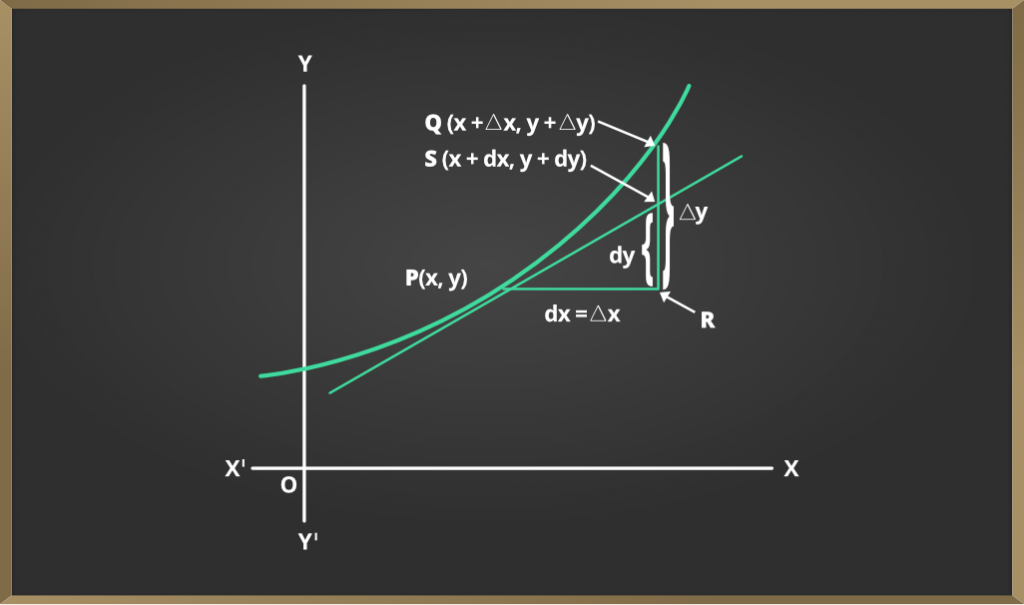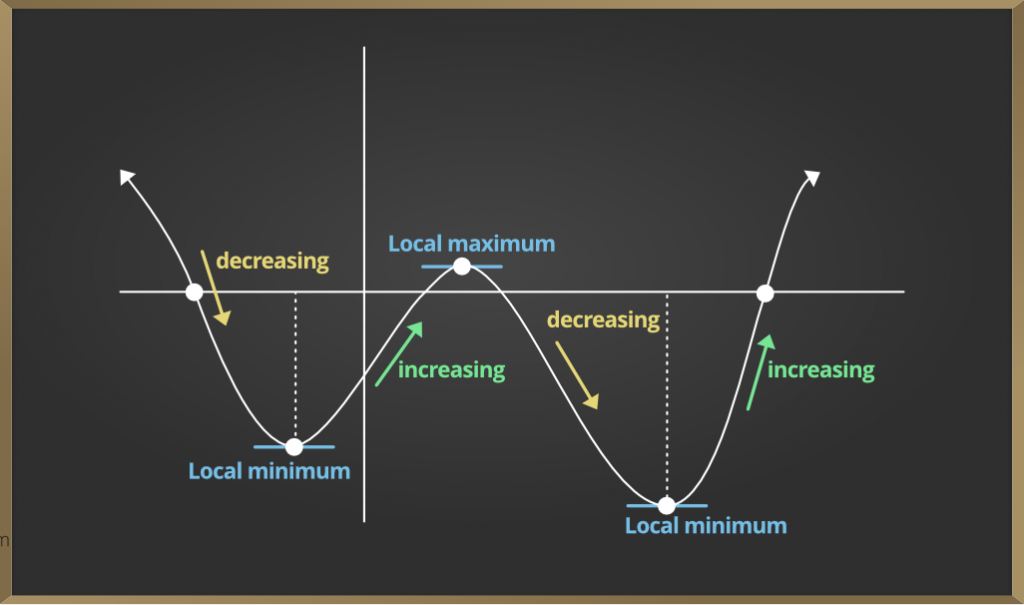GFG App
Open AppBrowser
Continue

# Approximations & Maxima and Minima – Application of Derivatives | Class 12 Maths

An approximation is similar but not exactly equal to something else. Approximation occurs when an exact numerical number is unknown or difficult to obtain. In Mathematics, we use differentiation to find the approximate values of certain quantities.

Let f be a given function and let y = f(x). Let ∆x denote a small increment in x.Now the increment in y like the increment in x, denoted by

∆y, is given by ∆y = f (x + ∆x) – f (x)

We define the following:

(i) dx (the differential of x) is defined by dx = ∆x.

(ii) dy (the differential of y) is defined by dy = f’(x) dx or dy = (dy/dx) * ∆x

If dx = ∆x is relatively small when compared to with x dy ≈ ∆y.

Example: Find the approximate value of √26.

Solution:

Here it is very easy to find the value of under root if the given number is perfect square but for such type of numbers we have to use the derivatives to find the approximate value of the function.

Let the f(x) =√x and the derivative of this is f’(x)= 1/2x^1/2

Now we know the formula of approximation

∆y ≈ ∆x = (dy/dx). ∆x f(x+∆x)- f(x) = f’(x). ∆x f(x+∆x)= f(x) + f’(x). ∆x

Here we will assume x near to 25 which is a perfect square.

So we will assume x = 25 x2 – x1 = 26 – 25 = 1

Here tells us the change in x. Let x = 25 and now we will put the values in the formula

f(x + ∆x) = f(x) + f’(x). ∆x f(25 + 1)

= f(25) + f'(25) f(26) = √25 + (1/2.25^1/2).1

=  5 + 1/10 √26

= 5 + 0.1

=  5.1

### Approximation and Errors

If we use the derivative of f(x) then this gives us the exact change in f(x) over the infinitely small interval dx. As we know the instantaneous rate of change is defined using the limit as a discrete value for a change in x so that ∆x goes to zero.

Example 1: Find the value of (8.01)4/3 + (8.01)2(8.01)4/3 + (8.01)2

Solution:

Let y = f(x) = x4/3 + x2y = f(x) = x4/3 + x2

Let x0 = 8 so that y0 = 16 + 64 = 80

Δx = 0.01 ⇒ Δy = f′(x) × Δx = (43 x 1/3 + 2x) × Δx = (83+16) × 0.01

=0.563=0.1867

⇒y0=y0+Δy

=80.1867

Example 2: Find the approximate value of f(3.02), where f(x) = 3x2 + 5x + 3.

Solution:

Let x = 3 and Δx = 0.02. Then,

since, f(3.02) = f(x + Δx) = 3(x + Δx)2 + 5(x + Δx) + 3

Note that Δy = f(x+Δx) – f(x).

Therefore,

f(x + Δx) = f(x) + Δy

≈ f(x) + f'(x)Δx                   (as ds = Δx)

f(3.02)  ≈ (3x2 + 5x + 3) + (6x + 5)Δx

= (27 + 15 + 3) + (18 + 5)(0.02)

= 45 + 0.46 = 45.46

Hence, the approximate value of f(3.02) is 45.46

### Maxima and Minima

Let f be a function defined on an interval I. Then

(a) f is claimed to possess a maximum value in I, if there exists some extent c in I such f(c) > f (x), for all x ∈ I.

The number f (c) is named the utmost value of f in I and therefore the point c is named some extent of the maximum value of f in I.

(b) f is claimed to possess a minimum value in I, if there exists some extent c in I such f (c) < f (x), for all x ∈ I.

The number f (c), during this case, is named the minimum value of f in I, and therefore the point c, during this case, is named some extent of minimum value of f in I.

(c) f is claimed to possess an extreme value in I if there exists some extent c in I such f (c) is either a maximum value or a minimum value of f in I.

The number f (c), during this case, is named an extreme value of f in I and therefore the point c is named an extreme.

### First Derivative Test

Let f be a function defined on an unbounded interval I and f be continuous at a juncture c in I. Then

(i) If f ′(x) changes sign from positive to negative as x increases through c, i.e., if f ′(x) > 0 at every point sufficiently on the brink of and to the left of c, and f ′(x) < 0 at every point sufficiently on the brink of and to the proper of c, then c may be a point of local maxima.

(ii) If f ′(x) changes sign from negative to positive as x increases through c, i.e., if f ′(x) < 0 at every point sufficiently on the brink of and to the left of c, and f ′(x) > 0 at every point sufficiently on the brink of and to the proper of c, then c may be a point of local minima.

(iii) If f ′(x) doesn’t change sign as x increases through c, then c is neither some extent of local maxima nor some extent of local minima. Such a point is called point of InflectionExample 1: Find all the points of local maxima and local minima of the function f(x) = 2×3– 6×2+ 6x +5.

Solution:

We have f(x) = 2×3 – 6×2 + 6x + 5 or f ′(x) = 6×2 – 12x + 6 = 6 (x – 1)2

or we can say, f ′(x) = 0 at x = 1

Thus, the only critical point of f is x = 1

Example 2: For the function, find the points of local maxima and local minima if any:

f(x) = -x3 + 3x2 – 3x

Solution:

The derivative is

f'(x) = -3(x-1)2

This will never be undefined, so x = 1 is the only critical point. Since (x – 1)2 is positive for all x ≠ 1, the derivative

f ‘(x) = -3(x – 1)2 is negative for all x ≠ 1.

Since f is decreasing, on both sides of number line, we have neither a minimum nor a maximum at x = 1.

### Second Derivative Test

Let f be a function defined on an interval I and c ∈ I. Let f be twice differentiable at c. Then

(i) x = c may be a point of local maxima if f ′(c) = 0 and f ″(c) < 0 the worth f (c) is local maximum value of f.

(ii) x = c may be a point of local minima if f c ′( ) 0 = and f ″(c) > 0 during this case, f (c) is local minimum value of f.

(iii) The test fails if f ′(c) = 0 and f ″(c) = 0. during this case, we return to the primary derivative test and find whether c may be a point of local maxima, local minima, or some extent of inflection.

The number f (c), during this case, is named an extreme value of f in I and therefore the point c is named an extreme.

Example: Find all the points of local maxima and local minima of the function f(x) = 2x3– 6x2+ 6x +5 if any.

Solution:

We have (x) = 2x3 -6x2 + 6x + 5 or

f'(x) = 6x2 – 12x + 6 = 6(x – 1)2

f”(x) = 12(x – 1)

Now f ′(x) = 0 gives x =1. Also f ″(1) = 0. Therefore, second derivative test fails here. So, we will consider the first derivative test

We have already seen in the above example that, using first derivative test, x =1 is neither a point of local maxima nor a point of local minima and so it is a point of inflection.

My Personal Notes arrow_drop_up